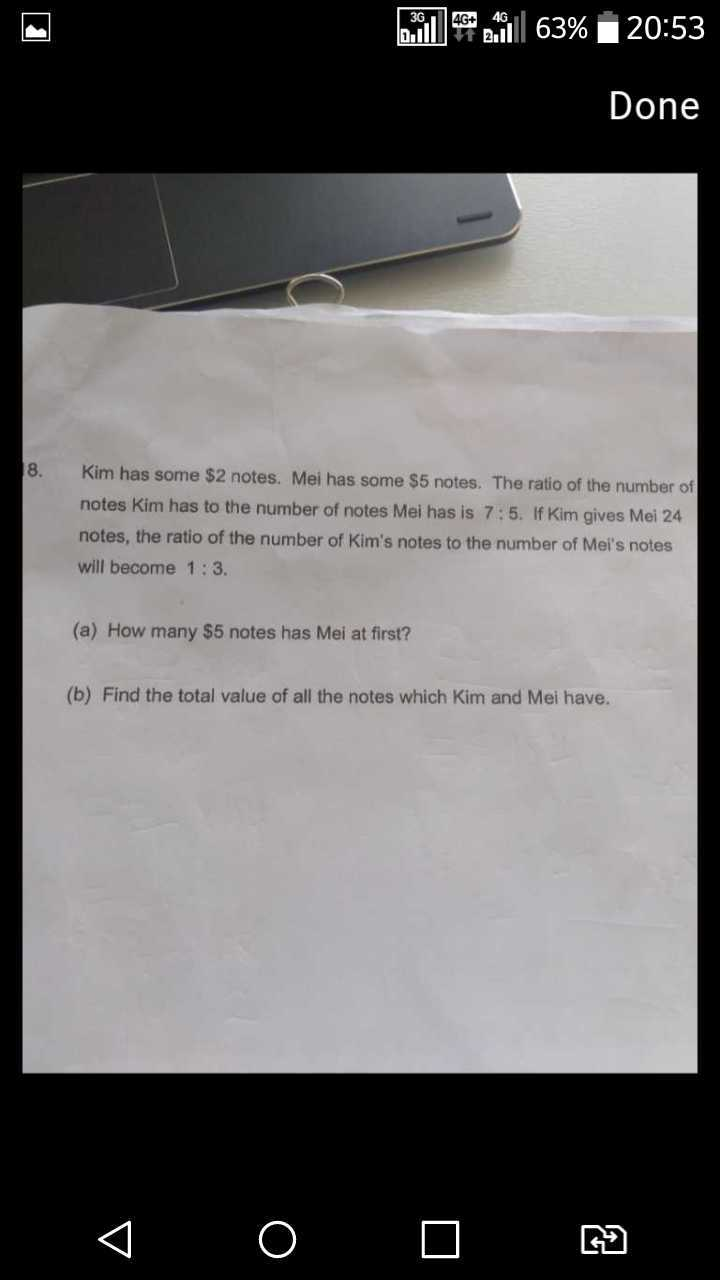# QuestionPls help. Thanks

2 Answers

# Answer

Use the Constant Total method.

Before and after the total is the same.

Before,
K : M : Total => 7 : 5 : 12

After
k : m: Total => 1 : 3 : 4 => 3 : 9 : 12 , now they share common units, u

7u – 3u = 4u = 24
u = 6

There are 5u of \$5, number of \$5 notes = 30 ## Answer for A

B) Number of \$2 notes => 7u = 42
Value of \$2 notes = 42 X 2 = \$84
Value of \$5 notes = 30 X 5 = \$150

Total value = 84 + 150 = \$234 #

0 Replies 0 Likes

What is 5u = 15 x 6 =90??

1 Reply 0 Likes